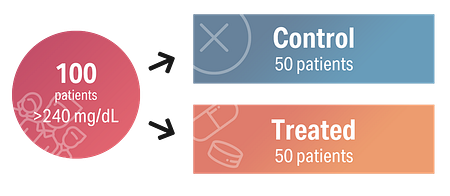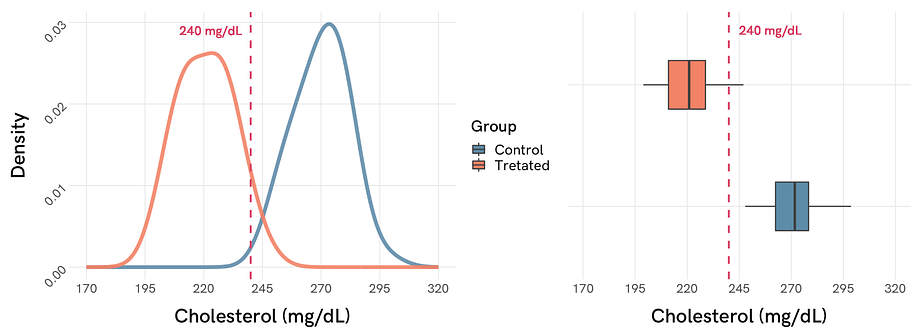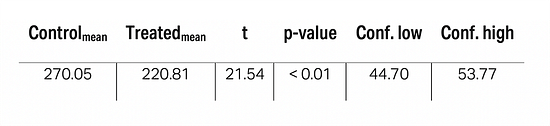Statistical jargon is plagued with technicalities and intricate mathematical notation. Throughout my experience in the biomedical field, I’ve encountered two types of people: those who feel an absolute fascination with this abstract language, and those who flee in terror every time they see formulas with a handful of Greek letters (although their studies are based on results from these formulas).

I work mostly for physicians and biomedical researchers with long-forgotten knowledge about applied statistics, so I end up spending more time answering questions of an interpretative nature than doing analyses. This is a direct consequence of how cryptic statistics can become even in their simplest manifestations.

One of the most frequently asked questions comes also from one of the most popular and commonly used statistical tests in medicine: the Student’s t-test and the p-value.

But what is Student’s t-test?

### Why use Student’s t-test

Given a continuous variable, Student’s t-test allows comparing means between 2 samples. Plain and simple, without going into major complications.

For those who like mathematical notation, Student’s t is equal to the sum of the means, divided by the combined standard deviation, and multiplied by the square root of 2 divided by the number of observations. It’s simpler than it sounds:

To illustrate Student’s t-test, we’ll design a small experiment. Suppose we want to carry out a modest clinical trial, in which we intend to evaluate whether a new drug reduces blood cholesterol levels in our patients after a month of use. To do this, we choose 100 individuals with hypercholesterolemia (>240 mg/dL) and assign them to one of the two treatment groups (control or treated), also randomly and in equal proportions. This way, we’ll have:Figure 1. Simple study design where 100 patients, whose cholesterol blood concentration is above 240 mg/dL, are randomly assigned to one of the two treatment groups: control or treated.

Once the study is finished, how can we know if the drug we’re testing has been effective enough? This is where Student t-test becomes helpful. But first, we must define what we believe will happen.

### Tell me about the possible hypotheses

It’s one of the things I say quite often: to correctly use statistics in medicine, a good previous experimental design is quintessential. The hypotheses must be defined before commencing any experiment. Why is it like this? Each statistical test evaluates different things differently. The most appropriate test to use will depend to a large extent, on how we formulate our initial hypotheses. It’s a common source of error that can lead to inconclusive results, or even worse, to erroneous conclusions!

Before starting a simple experiment like the one we designed above, we must define two hypotheses: the null (H₀) and the alternative (H₁).

A proper null hypothesis for this experiment could be defined by H₀: (x̅₁ - x̅₂) = 0 which, in our example means that there won’t be a difference in cholesterolemia when comparing the mean of the control group (x̅₁) with that of the treated group (x̅₂). This is intuitive: if there is no variation in the concentration of blood cholesterol, the means of control and treated group will be the same, right?

On the contrary, the alternative hypothesis can be defined by H₁: (x̅₁ - x̅₂) ≠ 0, which sets the opposite case to the previous one. If the drug we are testing causes a change in cholesterol levels, the difference in the means of both groups must be non-zero.

Null hypothesis (H₀): treatment has no effect on cholesterol levels.
Alternative hypothesis (H₁): treatment changes cholesterol levels.

These hypotheses set the ideal scenario to evaluate them using Student’s t-test, which specifically evaluates the difference between means. Once the hypotheses have been established, how different should these means be, so we can be sure that the new treatment is having an effect? In statistics, this is where the p-value comes in, but first, we‘ll explore our data visually and numerically.

### Look at your data!

It’s very common to store our measurements in spreadsheets, notebooks or databases. That’s where data lives, but we humans easily lose perspective once they reach a critical point of volume and density. Although in this example it’s perfectly possible to conclude with the naked eye, visually exploring the data is a highly recommended practice to have an impression of how each treatment group behaves.Figure 2. Density graph and boxplot representing the distribution of populations for the control group (in blue) and the treated group (in orange). The vertical dashed line (in red) shows the initial cholesterol and inclusion criteria for the study.

At first glance, we see that the center of the distributions of both groups is separated from each other along the abscissa axis, which means that, a priori, their means differ (later we’ll see if substantially enough). Besides, we can see that most of the individuals in the treated group are below the threshold of 240 mg/dL which, considering that before starting the clinical trial patients had a cholesterol concentration above that value, it’s an indicator of apparent improvement.

If we explore the measures of central tendency and dispersion in numerical form, we draw the same conclusions. Apparently, this new drug works!

But in science, and especially when working with people’s health, we can’t say that a treatment is effective based simply on the fact that two curves are displaced and separated by a vertical line. We have to resort to the objectivity of numbers.

### What is p-value

When physicians understand the true meaning of the p-value, there’s usually a paradigm shift in the way they interpret the results of their research. It should be clarified that Student’s t-test (and many more statistical tests) assume that the null hypothesis is true, which means that they start from the basis that the drug has no effect.

In technical terms, the p-value is the probability (from 0 to 1) of obtaining an effect as extreme as that observed in our data, assuming that the null hypothesis is true. For example, if we obtained a p-value of 0.6, there would be a probability of finding a difference between means such as we have observed in 60% of cases by mere chance. The scientific community agreed that a test is considered statistically significant when its p-value is below 0.05.

This is the key concept here: the p-value doesn’t provide any evidence about the certainty of the alternative hypothesis (which is what interests us), it just states that chance isn’t capable of explaining the variability we’ve observed in our data. This is where statistics ends and interpretation begins: how you would explain why the alternative hypothesis could be true?

### Interpreting the results

Before carrying out a Student t-test, and depending on the statistical software used, several doubts arise. Are my samples related? Should I assume equality of variances? One or two-tailed?

For the first question, and in our particular case, the two treatment groups we’ve defined are independent, since each group is made up of different people. It’d be the opposite case if we measured the cholesterol concentration on the first day of the clinical trial, before the administration of the drug, and re-measured the cholesterol after one month of treatment, to later analyze the differences that have occurred over time for the same patient. In that case, we should consider our measures as related. That’s why the design phase of the experiment is so important!

To check if the variances are equal between two groups, it’s necessary to perform a F-test, which we won’t discuss in this article. Broadly speaking, we can assume that variances are practically equal when the ratio between these approaches 1 (127/134 = 0.94). This influences Student’s t-test since if the variances were sufficiently different, we must use the Welch correction (that’s another article, too).

When Student t-test asks us if we want to perform the test one or two-tailed, basically it’s asking us if we know in which direction we expect the effect to alter our mean. In medicine, this translates into whether we expect the drug to increase or decrease the concentration of cholesterol, or if both could happen at the same time. In life sciences, there is usually some prior evidence of the expected effect of a drug before testing it in humans: in vitro, in animal experimentation, etc. For this, it’s rather common to perform a one-tailed test. But if we wanted to be more restrictive with Student’s t-test, and given that in our initial alternative hypothesis we established that there are differences (but not in which direction), we will use a two-tailed test.Table 2. Output of a Student two-tailed t-test for 2 independent samples, assuming equal variances.

The first thing we find again are the means of each treatment group. At first glance, there’s an absolute difference of 50 mg/dL. But is it relevant? The next parameter obtained is the t-value, which indicates that the difference in means observed is almost 22 times the size of the variability in our data. That’s a lot! This translates into a p-value lower than 0.01 which, if we recall the previous explanation, it’s indicating that we have a probability of less than 1% of finding in our patients a variability as extreme as we have observed, that could be explained by mere chance. With all of the above, we reject the null hypothesis and accept the alternative one.

The last two parameters are the confidence interval: its low and high limit. This tells us that, in the treated group, we have found a difference in cholesterol between 44.70 and 53.88 mg/dL compared to the control group. Here’s where physicists should start making clinical interpretations!

### So, the new drug is effective?

Well, statistics will never be able to answer that question, it just gives us clues that something is happening and that isn’t explained by chance. Here begins the interpretive part. It isn’t uncommon in medicine to find statistically significant but clinically irrelevant evidence. For this particular case, the average of our treated patients is 220 mg/dL, which is still above the limit of what’s clinically recommended (~200 mg/dL). Yes, they‘ve improved, but perhaps other drugs have a greater effect, with fewer adverse effects, and so on. And it may even happen that, despite having found significant differences, what we are seeing is due to other factors, such as a change in diet during the clinical trial, an increase in physical activity, or even genetic variants. As researchers, we have to consider all the possible options and test them before drawing conclusions.

The take-home message from this sample experiment should be: this treatment reduces cholesterol between 45 and 54 mg/dL, in patients with an initial concentration above 240 mg/dL.

Is this enough to justify its use? It depends on who you ask! Again, this is the interpretive part to which statistics cannot (nor should) respond.

### To conclude

This example is extremely simple (it comes from simulated distributions in R), but I think it tangibly illustrates a case in which Student’s t-test could be useful. In actual clinical practice, many more variables are taken into consideration and even their partial contributions, for which the Student’s t-test is insufficient. This is where the world of regression and mixed models come into play.

And remember: to report a Student’s t-test you must include all the parameters: p-value alone isn’t enough! There are countless papers in which only this last parameter is provided and, as we’ve explained, this says little to nothing about actual differences, nor does it allow us to get an idea of the size of the effect we’re observing. Give confidence intervals, please!